# Basic functions - math word problems

#### Number of problems found: 2467

• TestingStudents of high school have 10 points for each good solved task. The wrong answer is deducted by 5 points. After solving 20 tasks, student Michael had 80 points. How many tasks did he solve correctly and how many wrong?
• Similarity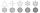The area of the regular 10-gon is 563 cm2. The area of similar 10-gon is 606 dm2. What is the coefficient of similarity.
• FloorThe floor area of ​​the room is 31 m2 and has a width of 4.3 m. How many centimeters of circumference measured the floor on the map at the scale 1:75?
• The dough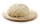The dough contains water, flour, and sugar. Water and flour in a ratio of 2: 3, flour, and sugar in a ratio of 2: 1. Determine the ratio of all three components of the dough.
• Age of familyThe age of father and son is in the ratio 10: 3. The age of the father and daughter is in a ratio of 5: 2. How old are a father and a son if the daughter is 20?
• The half lifeThe half-life of a radioactive isotope is the time it takes for a quantity of the isotope to be reduced to half its initial mass. Starting with 145 grams of a radioactive isotope, how much will be left after 3 half-lives?
• In the 14In the survey, the barangay had a certain number of families, and 60% of them are identified and belong to the marginalized sector's poor if the total families are 150. How many families belong to the poor sector?
• Force meterWe put the statuette on the force meter. The force meter showed a value 25 N. Then we placed the statuette on the force meter completely immersed in the water. The force meter showed a value 17 N. What is the volume of the statuette?
• Flowerbed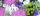On the flowerbed was planted 280 flowers - pansies and crayons. During the first week wilts quarter of pansies and an eighth crayons, which is 20% of all flowers. How many pansies were planted on the flowerbed?
• Collection of stampsJano, Rado, and Fero have created a collection of stamps in a ratio of 5: 6: 9. Two of them had 429 stamps together. How many stamps did their shared collection have?
• Rectangular fieldA rectangular field has a diagonal of length 169m. If the length and width are in the ratio 12:5. Find the dimensions of the field, the perimeter of the field and the area of the field.
• A five-digit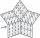A five-digit odd number has the sum of all digits (digits) five and contains two zeros. If we move each digit in the number one place to the left and move the first digit to the last place, we get a number 20,988 smaller. Find this unknown five-digit numb
• Brick weightThe brick weighs 2 kg and a half bricks. How much does one brick weigh?
• Bath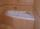In the bath is 30 liters of hot water. Then added 36 liters of cold water at temperature of 19 °C decreased temperature of water to 41 °C. What was the initial temperature of the hot water?
• Two doctorsDoctor A will determine the correct diagnosis with a probability of 93% and doctor B with a probability of 79%. Calculate the probability of proper diagnosis if both doctors diagnose the patient.
• SalesFrom statistics of sales goods, item A buy 51% of people and item B buys 59% of people. What is the probability that from 10 people buy 2 item A and 8 item B?
• Ratio of volumesIf the heights of two cylindrical drums are in the ratio 7:8 and their base radii are in the ratio 4:3. What is the ratio of their volumes?
• Unknown numberSamuel wrote unknown number. Then he had add 200000 to the number and the result multiply by three. When it calculated he was surprised, because the result would have received anyway, if write digit to the end of original number. Find unknown number.
• SaltSeawater containing 5% salt. How many kg of freshwater necessary to pour into 40 kg of seawater so that the salinity fell to 2%?
• Trio ratioHans, Alena and Thomas have a total of 740 USD. Hans and Alena split in the ratio 5: 6 and Alena and Thomas in the ratio 4: 5. How much will everyone get?

Do you have an interesting mathematical word problem that you can't solve it? Submit a math problem, and we can try to solve it.

We will send a solution to your e-mail address. Solved examples are also published here. Please enter the e-mail correctly and check whether you don't have a full mailbox.

Please do not submit problems from current active competitions such as Mathematical Olympiad, correspondence seminars etc...